# Chapter 12 Communication systems¶

## Ex 12_1 Pg-12.14¶

In :
from __future__ import division
Vm=40##peak value of the modulating signal
Vc=50##peak value of the carrier signal
m=Vm/Vc##modulation index
print "modulation index m=%.1f \n                 %%m=%.0f%% \n"%(m,m*100)

modulation index m=0.8
%m=80%



## Ex 12_2 Pg-12.14¶

In :
from math import sqrt, pi
L=40e-6#
C=12e-9#
x=2*pi*sqrt(L*C)#
fc=1/x##carrier  frequency
f=5e3##given audio frequency
fusb=fc+f##upper side band frequency
flsb=fc-f##lower side band frequency
BW=fusb-flsb##required bandwidth
print "Therefore upper side band frequency fusb=%.0f Hz \n"%(fusb)
print " Therefore lower side band frequency flsb=%.0f Hz \n"%(flsb)
print "\n required bandwidth BW=%.0fHz"%(BW)
#in the book fc is approximated to 230Khz but the exact answer is 229.72kHz

Therefore upper side band frequency fusb=234720 Hz

Therefore lower side band frequency flsb=224720 Hz

required bandwidth BW=10000Hz


## Ex 12_3 Pg-12.17¶

In :
from numpy import arange, pi, sqrt, sin
%matplotlib inline
from matplotlib.pyplot import plot, title, xlabel, ylabel, show, grid

# NOTE : Don't interrupt the kernel It will take time to output three modulated wave.

fm=5e3; #assume modulation frequency f=5kHz
fc=1080e3; #assume carrier frequency f=1080kHz
time=arange(0,8e-4,2.3148e-7)
#Waveform of modulated signal for m=0.75
m1=0.75; #modulation index
VmbyVc=m1
Vm=1; #we assume modulation voltage=1V
Vc=Vm/m1; #carrier voltage
k=VmbyVc; #modulation index = Vm/Vc
print "\n for modulation index m=0.75 Vc=%.2f V"%Vc

mt=k*sin(2*pi*fm*time);
sam =[]
for i in range(0,len(time)):
sam.append(Vc*(1+mt)*sin(2*pi*fc*time[i]))

plot(time[0:500],sam[0:500]);
title(' Waveform of modulated signal m=0.75');
xlabel('Time (sec)');
ylabel('Amplitude (Vc=1.33V)');

#xgrid(color("gray"));
show()

#Waveform of modulated signal for m=1
m1=1;
VmbyVc=m1
Vm=1; #we assume modulation voltage=1V
Vc=Vm/m1; #carrier voltage
k=VmbyVc; #modulation index = Vm/Vc
print "\n for modulation index m=1    Vc=%.2f V"%Vc

mt=k*sin(2*pi*fm*time);
sam =[]
for i in range(0,len(time)):
sam.append(Vc*(1+mt)*sin(2*pi*fc*time[i]))

plot(time[0:500],sam[0:500]);
title(' Waveform of modulated signal m=0.75');
xlabel('Time (sec)');
ylabel('Amplitude (Vc=1.33V)');

#xgrid(color("gray"));
show()

#Waveform for modulated signal for m=1.25
m1=1.25;
VmbyVc=m1
Vm=1; #we assume modulation voltage=1V
Vc=Vm/m1; #carrier voltage
k=VmbyVc; #modulation index = Vm/Vc
print "\n for modulation index m=1.25 Vc=%.2f V"%(Vc)

mt=k*sin(2*pi*fm*time);
sam =[]
for i in range(0,len(time)):
sam.append(Vc*(1+mt)*sin(2*pi*fc*time[i]))

plot(time[0:500],sam[0:500]);
title(' Waveform of modulated signal m=0.75');
xlabel('Time (sec)');
ylabel('Amplitude (Vc=1.33V)');

#xgrid(color("gray"));
show()

 for modulation index m=0.75 Vc=1.33 V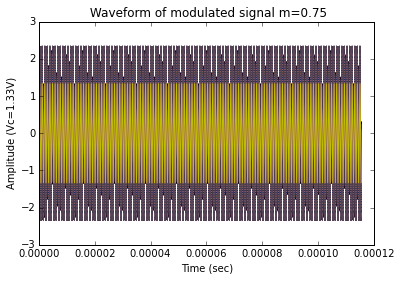for modulation index m=1    Vc=1.00 V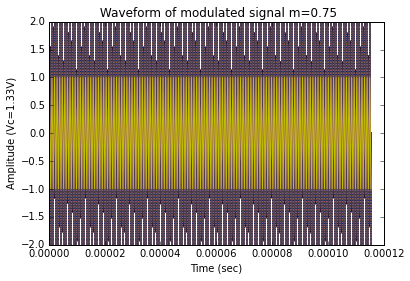for modulation index m=1.25 Vc=0.80 V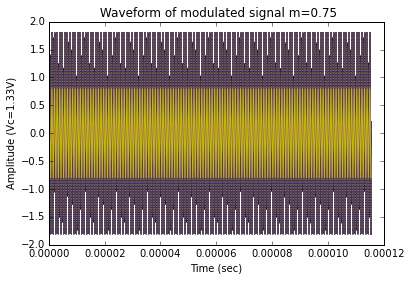## Ex 12_4 Pg-12.22¶

In :
from __future__ import division
from math import pi
#modulation index
Vm=10##peak value of the audio frequency signal
Vc=50##peak value of the carrier signal
m=Vm/Vc##modulation index
print "modulation index m=%.1f \n     ie          %%m=%.0f %% \n"%(m,m*100)
#sideband frequencies
wm=2*pi*500#
fm=wm/(2*pi)#
wc=2*pi*1e5#
fc=wc/(2*pi)#
fusb=fc+fm##upper side band frequency
flsb=fc-fm##lower side band frequency
print "\n Therefore upper side band frequency fusb=%.1f kHz \n"%(fusb*1e-3)
print " Therefore lower side band frequency fusb=%.1f kHz \n"%(flsb*1e-3)
#amplitude of each sinusoidal frequency
A=m*Vc/2#
print "\n amplitude of upper and lower side bands=%.f V \n"%(A)
#bandwidth required
BW=fusb-flsb##required bandwidth
print "\n required bandwidth BW=%.0fHz\n"%(BW)
P=Vc**2/(2*R)*(1+m**2/2)
print "\n power delivered to the load %.3f W \n "%(P)
#transmission efficiency
n=m**2/(2+m**2)*100#
print "\n transmission efficiency n=%.2f %% \n"%(n)

modulation index m=0.2
ie          %m=20 %

Therefore upper side band frequency fusb=100.5 kHz

Therefore lower side band frequency fusb=99.5 kHz

amplitude of upper and lower side bands=5 V

required bandwidth BW=1000Hz

power delivered to the load 2.125 W

transmission efficiency n=1.96 %



## Ex 12_5 Pg-12.23¶

In :
from __future__ import division
Pc=400##power of the carrier signal
m=0.8##modulation index
P=Pc*(1+m**2/2)
print "  Therefore total power in the modulated wave is %.0f W"%(P)

  Therefore total power in the modulated wave is 528 W


## Ex 12_6 Pg-12.23¶

In :
from __future__ import division
m=0.75##modulation index
P=20##total power in kW
Pc=P/(1+m**2/2)#since P=Pc*(1+m**2/2)
print "therefore carrier power in the modulated wave is %.1f kW"%(Pc)
Psb=Pc*m**2/4##side band power
Pusb=Psb#
Plsb=Psb#
print "\n Pusb=%.1f kW \n Plsb=%.1f kW"%(Pusb,Plsb)

therefore carrier power in the modulated wave is 15.6 kW

Pusb=2.2 kW
Plsb=2.2 kW


## Ex 12_7 Pg-12.25¶

In :
from __future__ import division
from math import sqrt
m=0.6##modulation index
Itotal=5##total antenna current
#Ic=total antenna current when only the carrier is sent
Ic=Itotal/sqrt(1+m**2/2)#since Itotal=Ic*sqrt(1+m**2/2)
print "Therefore total antenna current when only the carrier is sent Ic=%.1f A"%(Ic)

Therefore total antenna current when only the carrier is sent Ic=4.6 A


## Ex 12_8 Pg-12.26.¶

In :
from sympy import symbols, sqrt
Ic=symbols('Ic')##unmodulated carrier signal
Itotal=1.15*Ic##total rms current when the signal is modulated
x=Itotal/Ic#
y=2*((x)**2-1)
m=sqrt(y)
print "modulation index m=%.1f "%(m)

modulation index m=0.8


## Ex 12_9 Pg-12.28¶

In :
from math import sqrt
m1=0.6##first modulation index
m2=0.3##second modulation index
m3=0.4##third modulation index
mt=sqrt(m1**2+m2**2+m3**2)#
print "total modulation index m=%.3f "%(mt)

total modulation index m=0.781


## Ex 12_10 Pg-12.28¶

In :
from math import sqrt
from __future__ import division
Ptotal=11.8##radiated power in kW when the carrier is modulated
Pc=10##radiated power in kW when the carrier is unmodulated
m=sqrt(2*((Ptotal/Pc)-1))
#when another sine wave of 30% of the
#initial modulation is transmitted simultaneously then
m1=0.3##added sine wave signal is 30%
mt=sqrt(m1**2+m**2)#
print "total radiated power P=%.2f kW "%(P)

total radiated power P=12.25 kW


## Ex 12_11 Pg-12.30¶

In :
from __future__ import division
Ptotal=10##radiated power in kW when the carrier is modulated
m=0.75##modulation index
Pc=Ptotal/(1+m**2/2)#since Ptotal=Pc*sqrt(1+m**2/2)
print "\n carrier power Pc=%.1f kW \n"%(Pc)

 carrier power Pc=7.8 kW



## Ex 12_12 Pg-12.30¶

In :
from __future__ import division
fc=1000e3##carrier  frequency
fm1=300##first audio frequency
fm2=800##second audio frequency
fm3=1e3##third audio frequency
fusb1=fc+fm1##upper side band frequency
flsb1=fc-fm1##lower side band frequency
fusb2=fc+fm2##upper side band frequency
flsb2=fc-fm2##lower side band frequency
fusb3=fc+fm3##upper side band frequency
flsb3=fc-fm3##lower side band frequency
print "fusb1=%.1f kHz \n flsb1=%.1f kHz\n\n fusb2=%.1f kHz\n"%(fusb1*1e-3,flsb1*1e-3,fusb2*1e-3)
print " flsb2=%.1f kHz \n\n fusb3=%.1f kHz \n flsb3=%.1f kHz \n"%(flsb2*1e-3,fusb3*1e-3,flsb3*1e-3)

fusb1=1000.3 kHz
flsb1=999.7 kHz

fusb2=1000.8 kHz

flsb2=999.2 kHz

fusb3=1001.0 kHz
flsb3=999.0 kHz



## Ex 12_13 Pg-12.30¶

In :
from __future__ import division
from math import sqrt
m1=0.55##first modulation index
m2=0.65##second modulation index
mt=sqrt(m1**2+m2**2)#
Pc=360###power radiated by the carrier signal
print "Therefore total sideband power radiated Psb=%.3f W"%(Psb)
#in the question Pc is taken as 360W but in the answer it is taken as
#300W I have taken Pc=300W so that Psb=150.5W

Therefore total sideband power radiated Psb=130.500 W


## Ex 12_14 Pg-12.30¶

In :
from __future__ import division
from math import sqrt

m=0.5## modulation index
It=12##antenna current when AM transmitter is 50% modulated
Ic=It/sqrt(1+m**2/2)##carrier current
m=0.9##when modulation depth is increase to 0.9
It=Ic*sqrt(1+m**2/2)
print "Therefore total antenna current It=%.2f A"%(It)

Therefore total antenna current It=13.41 A


## Ex 12_15 Pg-12.31¶

In :
from __future__ import division
from math import sqrt
from sympy import symbols
## m1=0.6## modulation index
It=1.5##antenna current when AM transmitter is 50% modulated
Ic=It/sqrt(1+m1**2/2)##carrier current
m2=0.7#when another modulated signal is added
m=sqrt(m1**2+m2**2)##total modulation index
It=Ic*sqrt(1+m**2/2)

print "\n Therefore total antenna current It=%.2f A \n"%(It)
print "Ptotal=Pc+Pc*m**2/4+Pc*m**2/4 => Total power radiated"
Pc=symbols('Pc')#
Ptotal=Pc+Pc*m**2/4+Pc*m**2/4 #
P=Pc+Pc*m**2/4 #Total power if one of the side band is suppressed
per_P=P/Ptotal*100##percentage power saving
print "\n Therefore percentage power saving %%P=%.0f %%"%(per_P)

 Therefore total antenna current It=1.67 A

Therefore percentage power saving %P=89 %


## Ex 12_16 Pg-12.32¶

In :
from __future__ import division
from math import sqrt,pi

print " Output voltage of the transmitter Vam=400(1+0.4sin6280t)sin3.14*10**7t"
Vc=400##amplitude of carrier voltage
m=0.4##modulation index
wm=6280#
wc=3.14e7#
fc=wc/(2*pi)#
fm=wm/(2*pi)#
Pc=Vc**2/(2*R)#
Ptotal=Pc*(1+m**2/2)#
print "\n carrier frequency Fc=%.0f MHz \n modulating frequency =%.0f Hz \n "%(fc*1e-6,fm)
print "\n carrier power Pc=%.2f W \n Total power output Ptotal=%.1f W \n"%(Pc,Ptotal)
#peak power output results when modulating signal
#is at the peak of the +ve half cycle
Vm=m*Vc#
V=Vc+Vm##peak output voltage
P=V**2/(2*R)##peak power output
print "\n Peak output voltage P=%.2f W "%(P)
#The exact value of fc is 4997465 Hz but in the book the
#value is taken as 5 MHz  same is the case for fm

 Output voltage of the transmitter Vam=400(1+0.4sin6280t)sin3.14*10**7t

carrier frequency Fc=5 MHz
modulating frequency =999 Hz

carrier power Pc=133.33 W
Total power output Ptotal=144.0 W

Peak output voltage P=261.33 W


## Ex 12_17 Pg-12.33¶

In :
from numpy import arange
from math import pi,sin
from __future__ import division
%matplotlib inline
from matplotlib.pyplot import plot,title,show,grid,xlabel,ylabel
print " equation of the Am sine wave =Vc(1+m*sinwm*t)*sinwc*t"
Vc=12##amplitude of carrier voltage
m=0.5##modulation index
fc=10e6##carrier frequency
fm=1e3##modulated frequency
wc=2*pi*fc#
wm=2*pi*fm#
t=arange(0,8e-4,2.3148e-7)
Vam =[]
for tt in t:
Vam.append(Vc*(1+m*sin(wm*tt)*sin(wc*tt)))
fusb=fc+fm##upper side band frequency
flsb=fc-fm##lower side band frequency
A=m*Vc/2##amplitude of side bands

#plotting of the graph
x=[ flsb ,flsb  ]##x-coordinate
y=[  0   , A  ]##y-coordinate

plot(x,y)
x1=[10e6 , 10e6]##x-coordinate
y1=[ 0   ,  12]##y-coordinate
plot(x1,y1)
x2=[fusb , fusb]##x-coordinate
y2=[ 0   ,  A]##y-coordinate
plot(x2,y2)
x5=[flsb-1e3, flsb-1e3]##x-coordinate
y5=[    0   ,    4    ]##y-coordinate
plot(x5,y5)
x6=[fusb+1e3 ,fusb+1e3]##x-coordinate
y6=[  0      ,   4 ]##y-coordinate
plot(x6,y6)

xlabel('Frequency(Hz)')#
ylabel('amplitude of carrier signal(V)')#
title("Frequency Spectrum of AM wave")
grid()
show()

 equation of the Am sine wave =Vc(1+m*sinwm*t)*sinwc*t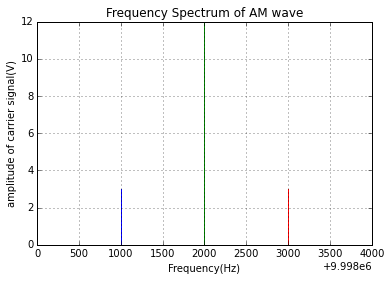## Ex 12_18 PG-12.18¶

In :
from sympy import symbols
print " When modulation index m=100%% "
m=1#
print " Now Pdsbfc=1.5*Pc or Pdsbfc/Pc=1.5"
Pc=symbols('Pc')
Pdsbfc=Pc*(1+m**2/2)##power required for double sideband with full carrier transmission
Pssb=Pc*m**2/4#
per_P=(Pdsbfc-Pssb)/Pdsbfc*100
x=per_P
print "  %%Power saving %%P :%f %%\n\n"%(x)

print " When modulation index m=50%% "
m=0.5#
Pdsbfc=Pc*(1+m**2/2)##power required for double sideband with full carrier transmission
print "  Now Pdsbfc=Pc*(1+m**2/2) \n  Pdsbfc=1.125*Pc  or Pdsbfc/Pc=1.125\n"
Pssb=Pc*m**2/4#
per_P=(Pdsbfc-Pssb)/Pdsbfc*100
x=per_P
print "  %%Power saving %%P :%.2f %%"%(x)

 When modulation index m=100%%
Now Pdsbfc=1.5*Pc or Pdsbfc/Pc=1.5
%Power saving %P :75.000000 %

When modulation index m=50%%
Now Pdsbfc=Pc*(1+m**2/2)
Pdsbfc=1.125*Pc  or Pdsbfc/Pc=1.125

%Power saving %P :94.44 %


## Ex 12_19 Pg-40¶

In :
from __future__ import division
R=2##frequency deviation constant in KHz/V
V=20##amplitude of the modulation signal
fd=R*V## frequency deviation
f=4##frequency applied in kHz
print "\n Therefore frequency deviation f=%.0f kHz \n"%(fd)
m=fd/f##modulation index
print "    modulation index m=%.0f "%(m)

 Therefore frequency deviation f=40 kHz

modulation index m=10


## Ex 12_20 Pg-40¶

In :
from __future__ import division
print "when modulating voltage V=2.5 V"
V=2.5##modulating voltage
fd1=5## frequency deviation in kHz
R=fd1/V##frequency deviation constant in KHz/V
print "\n frequency deviation constant R=%.0f KHz/V \n"%(R)
print "when modulating voltage V=7.5 V"
V=7.5##new value amplitude of the modulating voltage
fd2=R*V#new frequency deviation in kHz
print "\n Therefore frequency deviation f=%.0f kHz \n"%(fd2)
print "when modulating voltage V=10 V"
V=10##new value amplitude of the modulating voltage
fd3=R*V#new frequency deviation in kHz
print "\n Therefore frequency deviation f=%.0f kHz \n"%(fd3)
fm=0.5##modulation frequency in kHz ie 0.5kHz=500Hz
mf1=fd1/fm#
mf2=fd2/fm#
fm1=.25#new modulation frequency in kHz ie 0.25kHz=250Hz
mf3=fd3/fm1#
print " \n modulation index \n mf1=%.0f \n mf2=%.0f \n mf3=%.0f \n"%(mf1,mf2,mf3)

when modulating voltage V=2.5 V

frequency deviation constant R=2 KHz/V

when modulating voltage V=7.5 V

Therefore frequency deviation f=15 kHz

when modulating voltage V=10 V

Therefore frequency deviation f=20 kHz

modulation index
mf1=10
mf2=30
mf3=80



## Ex 12_21 Pg-41¶

In :
mf=60##modulation index
fm=0.4##modulation frequency in kHz ie 0.4kHz=400Hz
#fd_max=maximum frequency deviation
fd_max=mf*fm##since mf=fd_max_/fm
print "maximum deviation fd_max=%.0f kHz \n"%(fd_max)
V=2.4##modulating voltage
R=fd_max/V##frequency deviation constant in KHz/V
print "when modulating voltage V=3.2 V"
V=3.2#
fd=R*V##frequency deviation
fm=0.25#modulation frequency in kHz ie 0.25kHz=250Hz
mf=fd/fm#
print " \n modulation index mf=%.0f"%(mf)
#in the book the final answer for modulation mf=80
#is wrong the correct answer is mf=128

maximum deviation fd_max=24 kHz

when modulating voltage V=3.2 V

modulation index mf=128


## Ex 12_22 Pg-41¶

In :
R=5##frequency deviation constant in KHz/V
fm=10##modulation frequency in kHz
V=15##amplitude of the modulating signal
fd=R*V##frequency deviation
print "\n maximum frequency deviation  fd=%.0f KHz/V \n"%(fd)
mf=fd/fm#
print " \n modulation index mf=%.1f"%(mf)

 maximum frequency deviation  fd=75 KHz/V

modulation index mf=7.0


## Ex 12_23 Pg-41.43¶

In :
from __future__ import division
from math import pi,sqrt
print "equation of a frequency modulated v=A*sin(wc*t+mf*sin(wm*t))"
print "or v=A*sin(wc*t-fd/fm*cos(wm*t)) where fd=frequency deviation"
print "Now v=50*sin(5e8*t-10*cos(1000*t))"
A=50##peak value of the modulating signal
wc=5e8#
mf=10#
wm=1000#
fc=wc/(2*pi)##carrier frequency
print "\n carrier frequency fc=%.2f MHz \n"%(fc*1e-6)
fm=wm/(2*pi)##modulating frequency
print " modulating frequency fc=%.2f Hz \n"%(fm)
#fd_max=maximum frequency deviation
fd_max=mf*fm##since mf=fd_max_/fm
print " \n modulation index mf=%.0f"%(mf)
print "\n maximum deviation fd_max=%.2f Hz \n"%(fd_max)
Vrms=A/sqrt(2)##rms value of the modulating signal
R=75##wave resistance
P=Vrms**2/R#
print "\n Power dissipated by the wave in resistance P=%.2f W"%(P)

equation of a frequency modulated v=A*sin(wc*t+mf*sin(wm*t))
or v=A*sin(wc*t-fd/fm*cos(wm*t)) where fd=frequency deviation
Now v=50*sin(5e8*t-10*cos(1000*t))

carrier frequency fc=79.58 MHz

modulating frequency fc=159.15 Hz

modulation index mf=10

maximum deviation fd_max=1591.55 Hz

Power dissipated by the wave in resistance P=16.67 W


## Ex 12_24 Pg-41.47¶

In :
from __future__ import division
%matplotlib inline
from matplotlib.pyplot import plot,title,show,grid,xlabel,ylabel

V=5##amplitude of modulating voltage
R=1##frequency deviation constant in KHz/V
fd=V*R## frequency deviation in kHz
fm=15##modulating frequency in kHz
mf=fd/fm##modulation index
print " \n modulation index mf=%.3f"%(mf)#
print "Now we refer from the table-12.2 of Bessel function "
print " \n For modulation index mf=%.3f we take the value of J0,J1,and J2 "%(mf)#
J0=0.96##for carrier frequency
J1=0.18##first side frequency
J2=0.02##second side frequency
A=5#amplitude of the carrier frequency
J0=J0*A##for carrier frequency
J1=J1*A##first side frequency
J2=J2*A##second side frequency
print "\n J0=%.1fV\n J1=%.1fV\n J2=%.1fV "%(J0,J1,J2)
print "Now we plot the frequency spectrum"
x=[ 89.97,    89.97]##x-coordinate
y=[  0   ,    0.1]##y-coordinate
plot(x,y)
x1=[89.985,   89.985]##x-coordinate
y1=[  0   ,   0.9]##y-coordinate
plot(x1,y1)
x2=[90   , 90]##x-coordinate
y2=[ 0  , 4.8]##y-coordinate
plot(x2,y2)
x3=[90.015, 90.015]##x-coordinate
y3=[  0   ,  0.9]##y-coordinate
plot(x3,y3)
x4=[90.03, 90.03]##x-coordinate
y4=[  0   ,  0.1]##y-coordinate
plot(x4,y4)
x5=[90.04 ,90.04]##x-coordinate
y5=[  0   ,  5]##y-coordinate
plot(x5,y5)
x6=[ 89.96 , 89.96]##x-coordinate
y6=[  0     , 5]##y-coordinate
plot(x6,y6)
xlabel('Frequency(MHz)')#
ylabel('amplitude of carrier signal(V)')#
title("Frequency Spectrum")
grid()
show()


modulation index mf=0.333
Now we refer from the table-12.2 of Bessel function

For modulation index mf=0.333 we take the value of J0,J1,and J2

J0=4.8V
J1=0.9V
J2=0.1V
Now we plot the frequency spectrum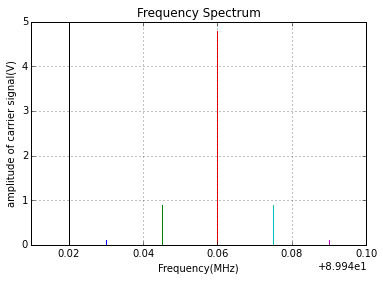## Ex 12_25 Pg-41.48¶

In :
from __future__ import division
fd=15##frequency deviation in kHz
f=75##maximum frequency deviation in kHz for FM broadcast
per_M=fd/f*100#
print "\n For FM broadcast percent modulation is %%M=%.0f%%\n"%(per_M)
f=25##maximum frequency deviation in kHz for TV broadcast
per_M=fd/f*100#
print "\n For TV broadcast percent modulation is %%M=%.0f%% \n"%(per_M)

 For FM broadcast percent modulation is %M=20%

For TV broadcast percent modulation is %M=60%



## Ex 12_26 Pg-41.48¶

In :
per_M=80## percent modulation in %
f=75##maximum frequency deviation in kHz for FM broadcast
fd=f*per_M/100##frequency deviation in kHz since %M=fd/f*100#
print "\n Therefore frequency deviation for FM broadcast fd=%.0f Khz \n"%(fd)
cs=2*fd##carrier swing
print " carrier swing=%.0f kHz\n"%(cs)
f=25##maximum frequency deviation in kHz for TV broadcast
fd=f*per_M/100##frequency deviation in kHz since %M=fd/f*100#
print "\n Therefore frequency deviation for TV broadcast fd=%.0f Khz \n"%(fd)
cs=2*fd##carrier swing
print " carrier swing=%.0f kHz\n"%(cs)

 Therefore frequency deviation for FM broadcast fd=60 Khz

carrier swing=120 kHz

Therefore frequency deviation for TV broadcast fd=20 Khz

carrier swing=40 kHz



## Ex 12_27 Pg-41.51¶

In :
fd=40##frequency deviation in kHz
cs=2*fd##carrier swing
print "\n carrier swing=%.0f kHz\n"%(cs)
fc=93.2e3###carrier frequency in kHz
fh=fc+fd##highest frequency reached
fl=fc-fd##lowest frequency reached
print "\n Therefore highest frequency reached fh=%.2f MHz\n "%(fh*1e-3)
print "Lowest frequency reached fl=%.2f MHz"%(fl*1e-3)
fm=5##modulating frequency in kHz
m=fd/fm##frequency modulation
print "\n\n modulation index m=%.0f "%(m)

 carrier swing=80 kHz

Therefore highest frequency reached fh=93.24 MHz

Lowest frequency reached fl=93.16 MHz

modulation index m=8


## Ex 12_28 Pg-41.51¶

In :
fc=50.4e3###carrier frequency in kHz
fh=50.405e3#highest frequency reached in kHz
fd=fh-fc##frequency deviation in kHz
print "\n Therefore frequency deviation produced fd=%.0f Khz \n"%(fd)
cs=2*fd##carrier swing
print "\n carrier swing=%.0f kHz\n"%(cs)
fl=fc-fd##lowest frequency reached
print "\n Therefore lowest frequency reached fl=%.3f MHz"%(fl*1e-3)

 Therefore frequency deviation produced fd=5 Khz

carrier swing=10 kHz

Therefore lowest frequency reached fl=50.395 MHz


## Ex 12_29 Pg-41.52¶

In :
cs=70##carrier swing in kHz
#since cs=2*fd
fd=cs/2##frequency deviation in kHz
fm=7#modulating frequency in kHz
m=fd/fm#
print "\n    Modulation index m=%.0f "%(m)

    Modulation index m=5


## Ex 12_30 Pg-41.52¶

In :
fh=99.047e3##highest frequency reached in kHz
fl=99.023e3##lowest frequency reached in kHz
fm=7#modulating frequency in kHz
cs=fh-fl##carrier swing
print "Carrier swing=%.0f kHz\n"%(cs)
fd=cs/2##frequency deviation in kHz
print "\n Therefore frequency deviation fd=%.0f Khz \n"%(fd)
fc=fh-fd###carrier frequency in kHz
print "\n Therefore carrier frequency fc=%.3f Mhz \n"%(fc*1e-3)
m=fd/fm#
print "\n Modulation index m=%.3f "%(m)

Carrier swing=24 kHz

Therefore frequency deviation fd=12 Khz

Therefore carrier frequency fc=99.035 Mhz

Modulation index m=1.714